Courses

# Test: Physics Mock- 5

## 45 Questions MCQ Test NEET Mock Test Series | Test: Physics Mock- 5

Description
This mock test of Test: Physics Mock- 5 for NEET helps you for every NEET entrance exam. This contains 45 Multiple Choice Questions for NEET Test: Physics Mock- 5 (mcq) to study with solutions a complete question bank. The solved questions answers in this Test: Physics Mock- 5 quiz give you a good mix of easy questions and tough questions. NEET students definitely take this Test: Physics Mock- 5 exercise for a better result in the exam. You can find other Test: Physics Mock- 5 extra questions, long questions & short questions for NEET on EduRev as well by searching above.
QUESTION: 1

Solution:
QUESTION: 2

Solution:
QUESTION: 3

### Four particles, each of mass 1kg, are placed at the corners of a square of side one metre in the XY-plane. If the point of intersection of the diagonal of the square is taken as a origin, the coordinates of the centre of mass are:

Solution:
QUESTION: 4

A bucket tied at the end of a 1.6 m long string is whirled in a vertical circle with cosntant speed. What should be the minimum speed so that the water from the bucket does not spill, when the bucket is at the highest position (Take g = 10 m/sec2)

Solution:
QUESTION: 5
A ball is dropped from a height h to the ground. If the coefficient of restitution is 'e' the height to which the ball goes up after it rebounds for nth time is
Solution:
QUESTION: 6
Three cells each of e.m.f. 1.5 V and internal resistance 1 Ω are connected in parallel. What would be the e.m.f. of the combination ?
Solution:
QUESTION: 7
Photons of energy 6eV are incident on a metal surface whose work function is 4eV. The minimum kinetic energy of the emitted photoelectrons will be
Solution:
QUESTION: 8
The working of a dynamo is based on principle of
Solution:
QUESTION: 9
In an oscillating LC circuit, the maximum charge on the capacitor is Q. The charge on the capacitor, when the energy is stored equally between the electric and magnetic field is
Solution:
QUESTION: 10
Five equal capacitors connected in series have a resultant capacitance of 4 $\mu$F. The total energy stored in these, when these are connected in parallel and charged to 400V is
Solution:
QUESTION: 11
A thin spherical conducting shell of radius R has charge q. Another charge Q is placed at the centre of the shell. The electrostatic potential at a point P at a distance R/2 from the centre of the shell is
Solution:
QUESTION: 12
Three similar cells, each of e.m.f. 2V and internal resistance r Ω send the same current through an external resistance of 2 Ω, when connected in series or in parallel. The strength of the current flowing through the external resistance is
Solution:
QUESTION: 13

Two trolleys of masses m and 3m are connected by a spring. They are compressed and released once. They move off in opposite directions and come to rest after covering distances S1 and S2 respectively. Assuming coefficient of friction uniform, the ratio of the distances S1  : S2 is

Solution:
QUESTION: 14
In a gravitational field, work done in transporting a mass from one point to another depends only upon
Solution:
QUESTION: 15

The distance of a planet from the sun is 5 times the distance between the earth and the sun. The time period of the planet is

Solution:
QUESTION: 16
In order that the heat flows from one part of a solid to another part, what is required
Solution:
QUESTION: 17
A gas exerts pressure on the walls of the container, because the gas molecules
Solution:
QUESTION: 18
The net charge on a current carrying conductor is
Solution:
QUESTION: 19
Two wires of equal length are placed perpendicular to the plane of paper at a distance of 5 cm from each other. If the currents flowing in first and second wires are 2 A and 5 A respectively in opposite direction, then the resultant magnetic field at mid-point of both the wires is
Solution:
QUESTION: 20
The magnetic lines of force inside a bar magnet
Solution:
QUESTION: 21

The Young's modulus of a wire of length (L) and radius (r) is Y N-m⁻2. If the length is reduced to L/2 and radius to r/2, then Young's modulus for the wire will be

Solution:
QUESTION: 22

The moment of inertia of a sphere of radius R and mass M about a tangent to the sphere is

Solution:
QUESTION: 23

A capillary tube when immersed vertically in a liquid records a rise of 3 mm. If the tube is immersed in the liquid at an angle of 60o with the vertical, then height of the liquid in the capillary tube will be

Solution:
QUESTION: 24

The acceleration \'a\' in m/s2 of a particle is given by a=3t2+2t+2 where t is the time. If the particle starts out with a velocity ν=2 m/s at t=0, then the velocity at the end of 2 second is

Solution:
QUESTION: 25
Which of the following does not represent a zero vector?
Solution:
QUESTION: 26

A particle moves towards east with velocity 5 m/s. After 10 seconds its direction changes towards north with same velocity. The average acceleration of the particle is

Solution:

correct option is B.

Intial velocity=v= 5m/seast wards

then after time t = 10sec

v= 5m/s north wards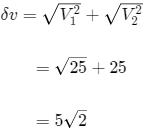acceleration, a = velocity / time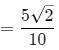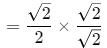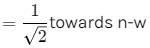QUESTION: 27
If a bullet of mass 5 gm moving with velocity 100 m/sec, penetrates the wooden block upto 6 cm. Then the average force imposed by the bullet on the block is
Solution:
QUESTION: 28
The normal magnifying power of a simple microscope is 6. The focal length of the convex lens used is
Solution:
QUESTION: 29
A system exhibiting simple harmonic motion must possess
Solution:
QUESTION: 30

Two stones are projected with the same speed but making different angles with the horizontal. Their horizontal ranges are equal. The angles of projection of one is π/3 and the maximum height reached by it in 102 metres. Then the maximum height reached by the other in metres is

Solution:

As ranges are equal, the angles of projection are θ and 90 - θ
One angle is 60o
∴ the other angle is 30o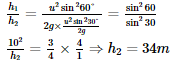QUESTION: 31
In spectral radiancy curve for cavity radiation, the wave-length of the maximum spectral rediancy
Solution:
QUESTION: 32
A stone is tied up to the end of a string and whirled in a vertical circle. The tension in the string is T1 when the stone is at the highest point and T2 when it is at the lowest point. Then
Solution:
QUESTION: 33
Given P=0.0030 m, Q=2.40 m and R=3,000 m, the number of significant figures in P, Q and R are respectively
Solution:
QUESTION: 34
A drop of oil is placed on the surface of water. Which of the following statement is correct?
Solution:
QUESTION: 35
Which of the following is a scalar quantity?
Solution:
QUESTION: 36

Two resistance thermometers are made, one of the platinum wire and the other of germanium wire. The resistance of both the thermometers are equal at room temperature. The two resistance wires are connected to a battery. Now the two resistance wires are heated to 100oC. The potential drop in the two resistance is measured. Which of the following statement is correct

Solution:

With rise in temp, the resistance of platinum wire increases but of germanium wire decreases.
Since the current is same in the wires, when connected in series, therefore heat produced ∝ R

QUESTION: 37
Faraday's 2nd law states that mass deposited on the electrode is directly proportional to
Solution:
QUESTION: 38

540 g of ice at 0oC is mixed with 540 g of water at 80oC. The final temp. of the mixture in oC will be

Solution:
QUESTION: 39

If 1 kg of ice at 0oC is mixed with 5 kg of water at 10oC, the fall in temperature of the mixture is (Take latent heat of ice=336 kJ-kg⁻1 and specific heat of water = 4.2 kJ-kg⁻1-oC⁻1)

Solution:
QUESTION: 40

The units of modulus of rigidity are

Solution:
QUESTION: 41
Which of the following is not a unit of time?
Solution:
QUESTION: 42
Bubble's of colourless soap solution appear coloured in sun light. This is due to
Solution:
QUESTION: 43
The Doppler's effect is applicable for
Solution:
QUESTION: 44
A body of mass (a), moving with a velocity (b) strikes another body of mass (c) at rest and gets embedded into it. The velocity of the system after collision is
Solution:
QUESTION: 45
The earth's radius is R and acc. due to gravity at its surface is g. If a body of mass m falls from a height h = R/5 from earth's surface, its potential energy decreases by
Solution: